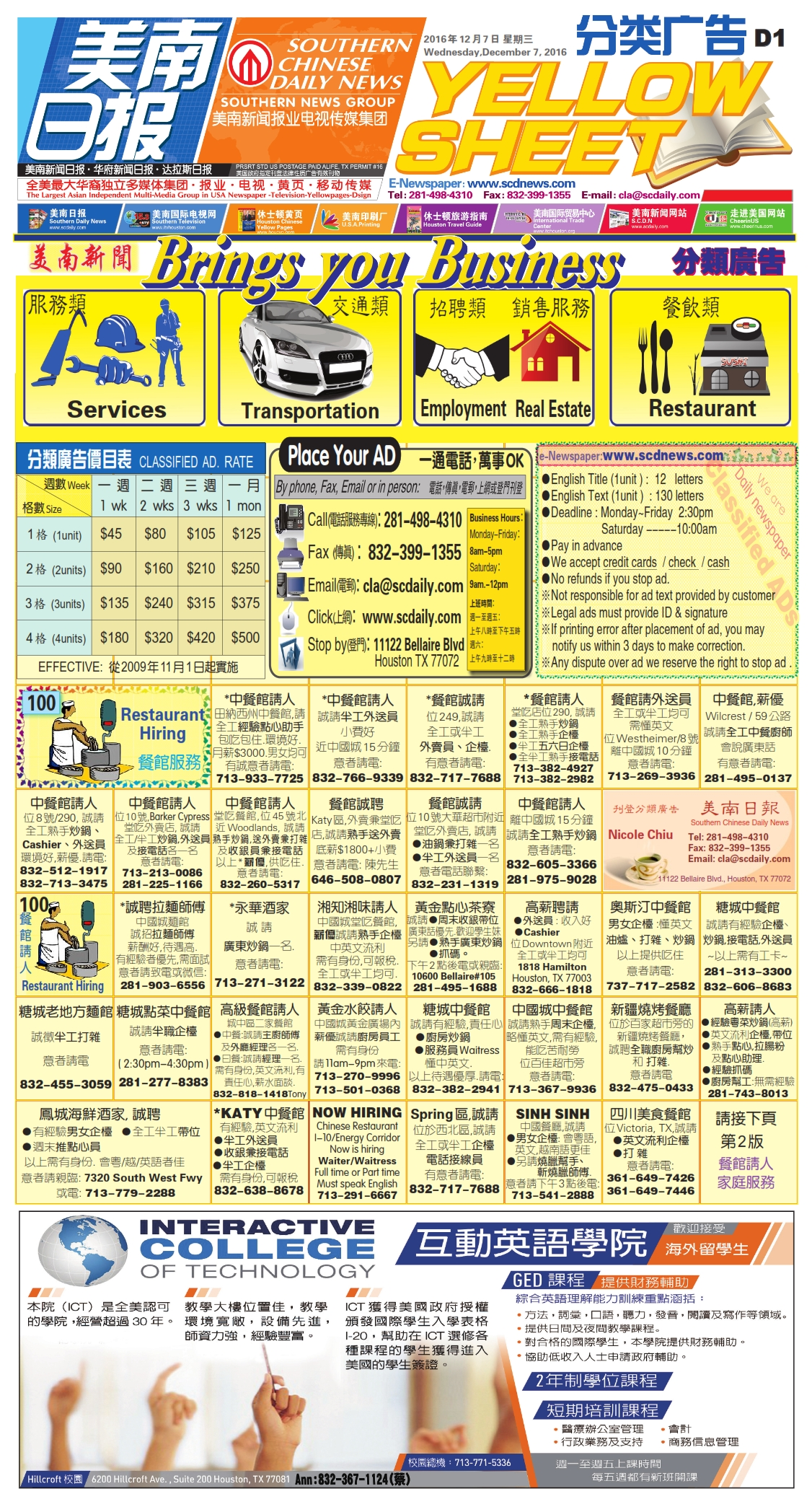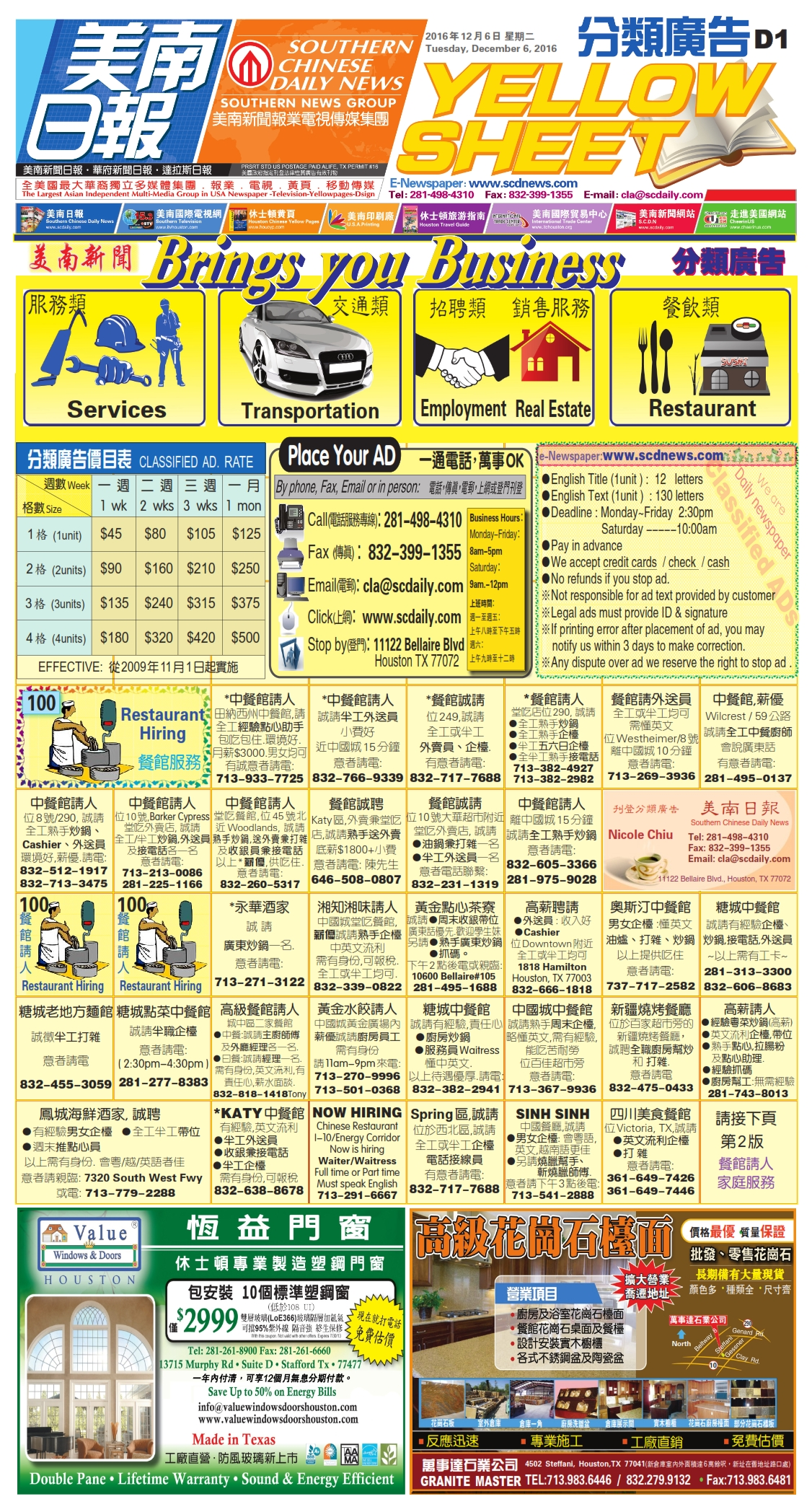161210 Epaper

 A Section B SectionC Section D Section161209 Epaper

 A Section B SectionC Section D Section161208 Epaper

 A Section B SectionC Section D Section161207 Epaper

 A Section B SectionC Section D Section161206 Epaper

 A Section B SectionC Section D Section161205 Epaper

 A Section B SectionC Section D Section161204 Epaper

 A Section B SectionC Section D Section161203 Epaper

 A Section B SectionC Section D Section161201 Epaper

 A Section B SectionC Section D Section161202 Epaper

 A Section B SectionC Section D Section161130 Epaper

 A Section B SectionC Section D Section